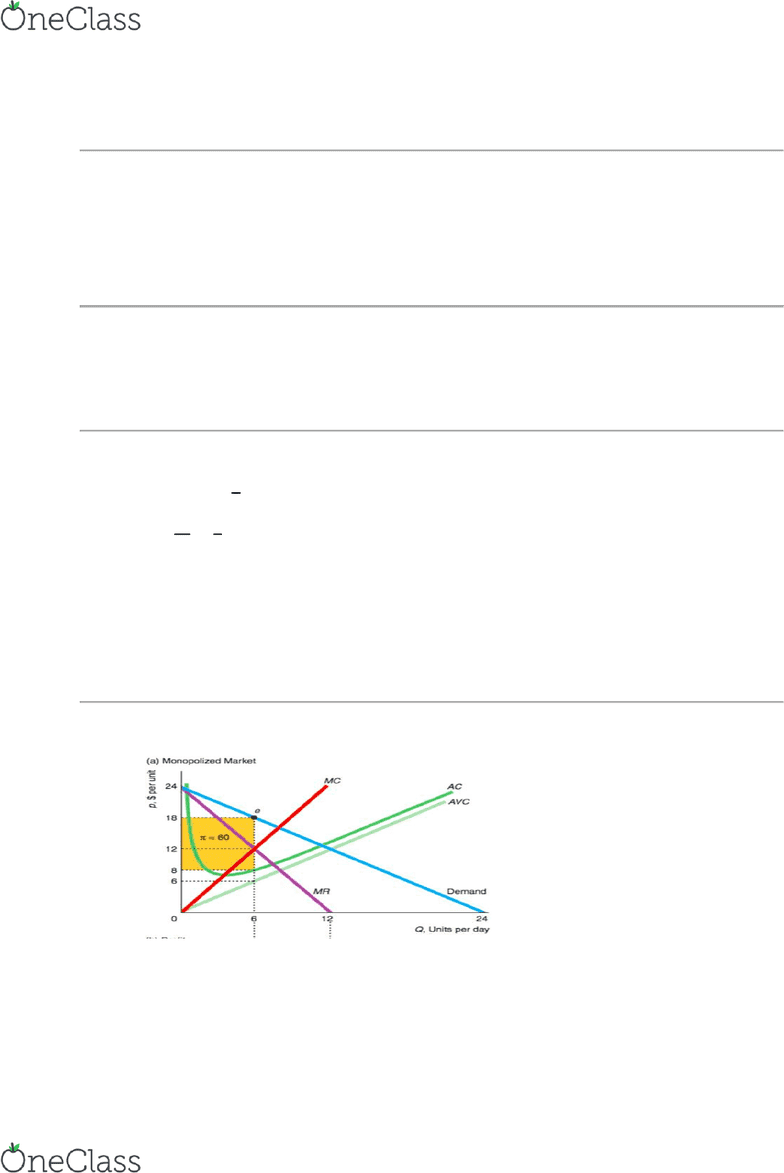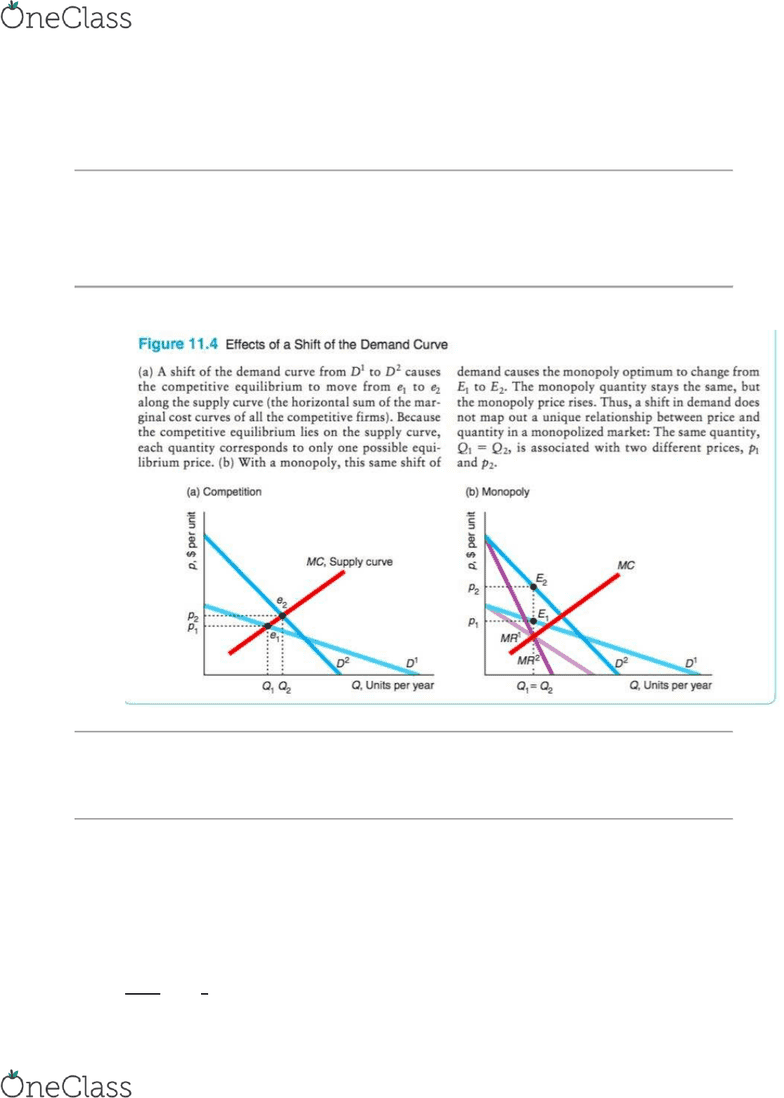Textbook Notes (270,000)
CA (160,000)
McGill (5,000)
ECON (300)
idk (2)
Chapter 11

# ECON 230D2 Chapter 11: Notes

Department
Economics
Course Code
ECON 230D2
Professor
idk
Chapter
11

This preview shows pages 1-2. to view the full 6 pages of the document.Chapter 11
Monopoly
o Occurs when there is only one firm dominating the market
o Other firms cannot enter
o Price setter
MR and price
o R = p*q
o MR = R’
MR and price elasticity of demand
o MR =  
o E = 

o Inelastic -1 < e <0
o Elastic e < -1
o Perfectly elastic e = -inf
o Perfectly inelastic e = 0
Price maximization
o
o Shutdown decision
o A monopoly shuts down to avoid making a loss in the short run if its price
is below its average variable cost at its profit maximizing quantity
find more resources at oneclass.com
find more resources at oneclass.com

Only pages 1-2 are available for preview. Some parts have been intentionally blurred.o In a long run, the monopoly shuts down if the price is less than its average
cost
Mathematical approach
o Profit maximizing point
o MR = MC
Effects of a shift of the demand curve
o
Market power
o the ability to a firm to charge a price above marginal cost and earn positive profit
Lerner index
o The ratio of the difference between price and marginal cost to the price:
(P-MC)/p
o if the firm is maximizing its profit, we can express the Lerner Index in terms of the
elasticity of demand by rearranging previous equation
o 
  
find more resources at oneclass.com
find more resources at oneclass.com